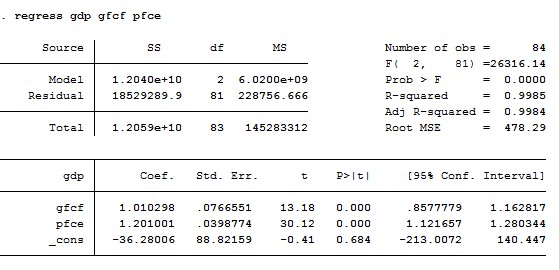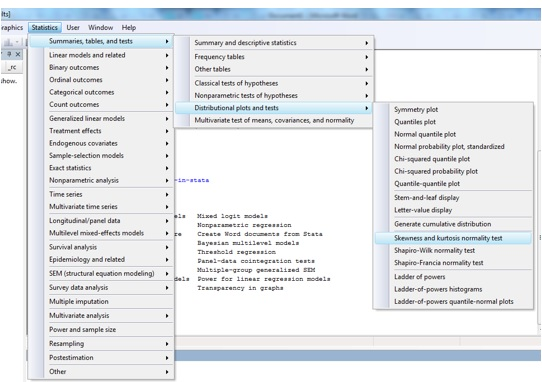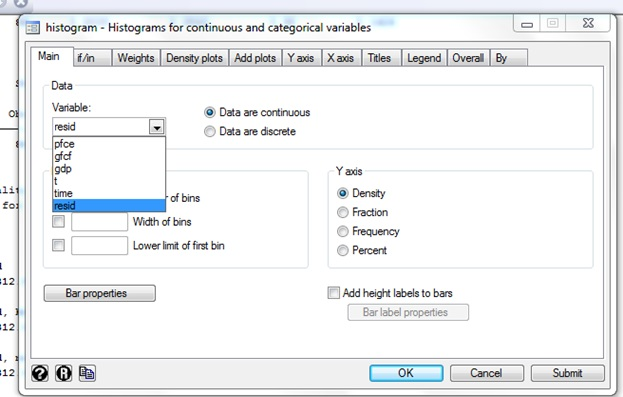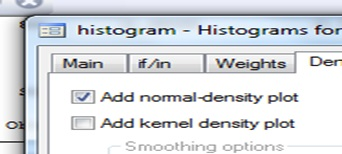# How to test normality in STATA?

The preceding articles showed how to conduct time series analysis in STATA on a range of univariate and multivariate models including ARIMA, VAR (Lag selection and stationarity in VAR with three variables in STATA) and VECM (VECM in STATA for two cointegrating equations). Time series data requires some diagnostic tests in order to check the properties of the independent variables. This is called ‘normality’. This article explains how to perform normality test in STATA.

Normality test helps to determine how likely it is for a random variable underlying the data set to be normally distributed. There are several normality tests such as Skewness Kurtosis test, Jarque Bera test, Shapiro Wilk test, Kolmogorov-Smirnov test and Chen-Shapiro test. This article shows two tests; Skewness Kurtosis and Jarque Bera tests because they are simple and popular.

This article uses quarterly data of the following variables of Indian economy for the time period 1997- 2018:

• Gross Domestic Product (GDP) (dependent variable).
• Gross Fixed Capital Formation (GFC) (independent variable).
• Private Final Consumption Expenditure (PFC) (independent variable).

The first step is to set the time series data. Thereafter proceed to the regression analysis.

## Regression analysis

The time series result will identify the residuals from the regression analysis.Figure 2: Regression results of the dataset

Next, use the below command in order to generate the residuals in the data set.

`regress gdp gfcf pcfe`

In order to predict the residuals from the regression model, use the below command.

`predict resid, residuals`

The command ‘predict’ helps in generating new variables (here it is resid or the error term in the model). This will create a new variable ‘resid’ in the data editor (figure below).Figure 3: Changes in data set after predicting regression residuals for performing normality in STATA

## Conducting normality test in STATA

In order to generate the distribution plots of the residuals, follow these steps (figure below):

• Go to the ‘Statistics’ on the main window
• Choose ‘Distributional plots and tests’
• Select ‘Skewness and kurtosis normality tests’.Figure 4: Procedure for Skewness and Kurtosis test for normality in STATA

After performing the above procedure, ‘sktest – Skewness and kurtosis test for normality’ box will appear (figure below). Select the main variable to test for normality (here it is ‘resid’).Figure 5: Selection of variable for Skewness and Kurtosis test for normality in STATA

This will give the following results (figure below). The null and alternative hypotheses for the normality test are:

• Null hypothesis: The data follows a normal distribution.
• Alternative hypothesis: The data does not follow a normal distribution.

## Skewness Kurtosis test for normality

Skewness is a measure of the asymmetry of the probability distribution of a random variable about its mean. It represents the amount and the direction of skew.  On the other hand, Kurtosis represents the height and sharpness of the central peak relative to that of a standard bell curve. The figure below shows the results obtained after performing Skewness and Kurtosis test for normality in STATA.Figure 6: Result of Skewness and Kurtosis Test for normality in STATA

‘sktest’ shows the number of observations (which is 84 here) and the probability of skewness which is 0.8035 implying that skewness is asymptotically normally distributed (p-value of skewness > 0.05). Similarly, Pr(Kurtosis)  indicates that kurtosis is also asymptotically distributed (p-value of kurtosis > 0.05).  Finally, chi(2) is 0.1426 which is greater than 0.05 implying its significance at 5% level. Consequently, the null hypothesis cannot be rejected. Therefore, according to Skewness test for normality, residuals show normal distribution.

## Jarque Bera test for normality

The other test of normality is Jarque Bera test. In order to perform this test, use the command ‘jb resid’ in the command prompt.

`jb resid`

The results will appear (figure below).Figure 7: Results for Jarque Bera test for normality in STATA

If p-value is lower than the Chi(2) value then the null hypothesis cannot be rejected. Therefore residuals are normality distributed.

As per the above figure, chi(2) is 0.1211 which is greater than 0.05. Therefore, the null hypothesis cannot be rejected. Moreover, there is no violation of the normal distribution assumption of error terms as the residuals are coming out to be normal.

## Normality through histogram

A histogram plot also indicates normality of residuals. A bell-shaped curve shows the normal distribution of the series. In order to generate the histogram plot, follow the below procedure.

• Go to ‘Graphics’ on the main bar.
• Select ‘histogram’.

The below figure will appear.Figure 9: Procedure for generating histogram plot for checking normality in STATA

Then choose the main variable and choose ‘Density’ under the Y-axis section. Click on ‘OK’ (figure below).Figure 10: Selecting variable in a histogram for checking normality in STATA

The below window will appear. Click on ‘Add normal density plot’.Figure 11: Procedure for generating a histogram for checking normality in STATA

Finally, click on ‘OK’ to generate the histogram plot showing normality distribution of the residuals (figure below).Figure 12: Histogram plot indicating normality in STATA

The figure above shows a bell-shaped distribution of the residuals. X-axis shows the residuals, whereas Y-axis represents the density of the data set. Thus this histogram plot confirms the normality test results from the two tests in this article.

The next article discusses the tests for heteroscedasticity. Heteroscedasticity is a violation of an important ordinary least squares (OLS) assumption that all residuals belong to a population that has a constant variance (homoscedasticity).

### Rashmi Sajwan

Research Analyst at Project Guru
Rashmi has completed her bachelors in Economic (hons.) from Delhi University and Masters in economics from Guru Gobind Singh Indrapastha University. She has good understanding of statistical softwares like STATA, SPSS and E-views. She worked as a Research Intern at CIMMYT international maize and wheat improvement centre. She has an analytical mind and can spend her whole day on data analysis. Being a poetry lover, she likes to write and read poems. In her spare time, she loves to do Dance.

#### Latest posts by Rashmi Sajwan (see all)

Discussions

1.Kofi Sefa-Boakye